| TOP | STORE | DSSF3 | Mmlib | Support | Contact Us |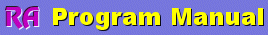| Japanese | English|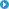About this manualIntroduction of RAOperation GuideImpulse responseRunning ACFReference ManualAppendix

Appendix of RA's program manual

Input and output device selection

An input device selection in RA is being interlocked with the volume control of Windows. So, after this volume is adjusted, volume control of Windows may not be changed.

However, selection or volume control of an output device cannot be set up here. It is because volume control is separately required for each output. It is necessary to set these up directly in the Windows volume control.

Caution: don't select a microphone by the output device. If you do so, the microphone input will be outputted directly and howling will be caused.

Peak level monitor, Input monitoring and output monitoring function

Input monitoring function

A peak level monitor supervises the digitized value of the input signal after A/D converted. Volume is displayed in dB relative to the maximum volume of the sound device as 0 dB. If an input device check box is checked, an input signal is calculated from the output of a mixer and the peak level can be supervised. The signal is distorted if this level is reached to 0 dB. In order to avoid a distortion, this level should be adjusted to about -5 dB generally. But, this level is dependent on the performance of an analog sound circuit. It is necessary to check it by using a test signal and an oscilloscope.

Output monitor function

The output level expresses the peak level of the digital outputs. Actually, the peak value of the mixer output is displayed. This signal is inputted into a D/A converter, going through the built-in analogue amplifier, then outputted from the line-out or the a headphone output.

This output level does not change even if the reproduction volume is adjusted in the Windows volume control. This output level can be adjusted only by the “Digital Output” in the signal generator. To output the signal correctly, the performance of the built-in analog amplifier becomes a problem. If the amplifier is not distorted to a full range signal, the signal generator can be used with the maximum digital volume. It seems that, however, the common built-in amplifier does not have such a good performance. So, you have to use it in the range in which the signal is not distorted.

Amplifier exists also after the usual output volume. To check the proper level of this amplifier, you need to measure the line out signal connected to another PC’ s line in by using the oscilloscope. In such a measurement, two PC are required (one is for generating a signal, and the other is for measuring that signal). This measurement is for the adjustment of the built-in amplifier of the PC, but the same procedure can be applied for the measurement of the general audio amplifier.

Once you adjust the digital output, the setting can always be used when using a signal generator. PC is used as a measuring instrument, but it is also an audio set simultaneously. It also becomes a noise level meter with the microphone. CD and DVD are also equipped. So, it can be said that the measurement of a PC will measure the performance of all equipment (if it says strictly). Conversely, it can be said, "All measurement can be performed if measurement of a PC can be performed correctly."

Time window in the FFT analyzer

The time window is used to decrease extra frequencies because frequency response is sometimes different because of a period with which a processed signal is taken out. When there is a portion that rapidly changes around the edge of the period taken out, it may influences the frequency response very much. Therefore, it is desired that the difference of the frequency response that depends on the chosen period is as small as possible. This "Time window" function prevents occurrence of high frequency components which is not included originally. As a shape of decrease is different by a kind of time window, it should be chosen properly. But in most cases, default setting of Hanning window is appropriate.

About volume control setting

The figure below is an illustration of the functional composition inside a PC.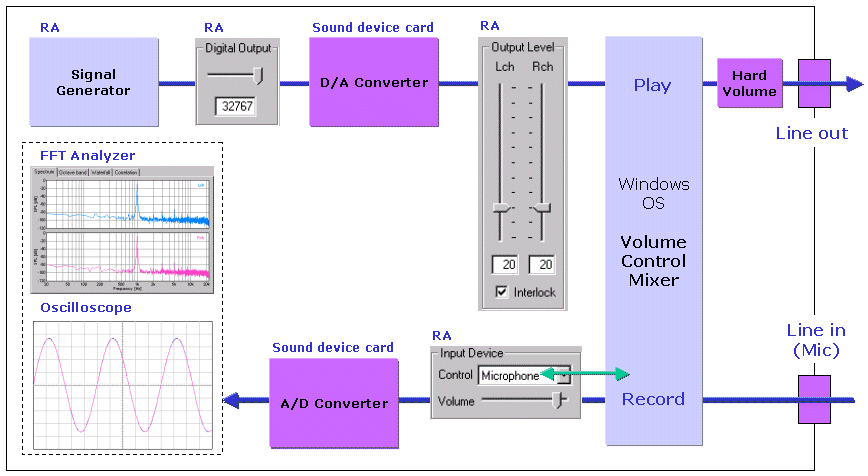The volume of the input device in RA controls the Windows volume control (recording). This is called "software volume."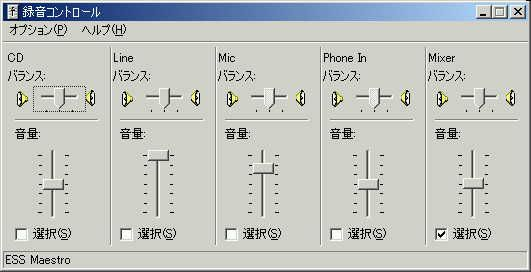Volume control of Windows should be silent except WAVE.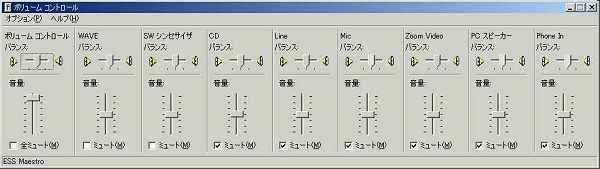The mechanical volume attached to the computer is called "hardware volume". In the case of DELL INSPIRON 7500, 3 in this figure is called a "sound volume dial".Test signal CD

For the accurate measurement, the signal CD is convenient. Clear test signals (without distortion, and with flat frequency characteristic) can be obtained easily. We recommend you to purchase this kind of CD even if you are using the DSSF3.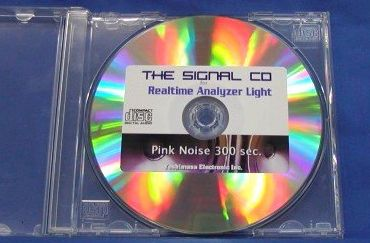Time resolution and frequency resolution in the FFT analyzer

In the FFT analyzer, the input wave data is extracted for every FFT size, then calculated, and the result is displayed one by one.

Display interval, or time resolution [s] is calculated as FFT size / sampling rate.

Frequency resolution [Hz] is its reciprocal and calculated as sampling rate / FFT size.

The time resolution and the frequency resolution could not be improved simultaneously. To solve the problem, FFT calculation is not performed for every FFT size, but performed with shifting data at a shorter interval. Consequently, the apparent time resolution can be improved with the frequency resolution maintained.

However, when the time resolution is raised, the number the FFT calculation within unit time and display processing will increase, and so the CPU load will much increased. Set the time resolution, looking at the rate of CPU use by the task manager of Windows.

New: In the RA Version 5.0.3.4 or later, the maximum time resolution of 8x is available.

About the correlation function

The cross-correlation function is used to investigate the similarity of two time series signals x(t) and y(t). Generally, it is expressed as the function of t (delay time) to show the time change of the similarity. Especially, the correlation function that shows the similarity of the signal itself is called the auto-correlation function (ACF). The ACF is a useful tool in signal analysis because it can be used to detect the periodicity of the signal. The ACF is defined as the following equation. In the equation, x(t) is the input signal, t is the delay time, and 2T is the integration time. The running ACF measured in RA is the short term ACF, which is calculated at a short interval with shifting the input data.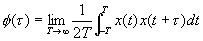In RA and SA, three parameters can be set. The first is Integration time and the second is Max delay time (in RA, it is called Time range). These parameters decide how to calculate the ACF for each data frame. The illustration below shows the calculation process of the running ACF for two successive data frames. A portion of the input signal (C-D) is shifted, multiplied with the original signal (A-B), and summed up to calculate the correlation value at each sample (t). The max delay time decides the time range of t in which the ACF is calculated. When the max delay time is longer than the integration time, the ACF is calculated up to the max delay time. When the max delay time is shorter than the integration time, the ACF is calculated up to the max delay time too. The third parameter, Running step decides the calculation interval of the running ACF.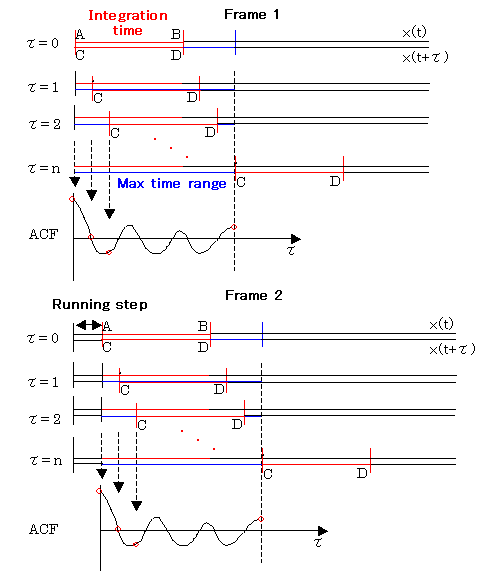Y Store.About this manualIntroduction of RAOperation GuideImpulse responseRunning ACFReference ManualAppendix

| TOP | STORE | DSSF3 | RAE | RAD | RAL | MMLIB | Support | Contact Us |